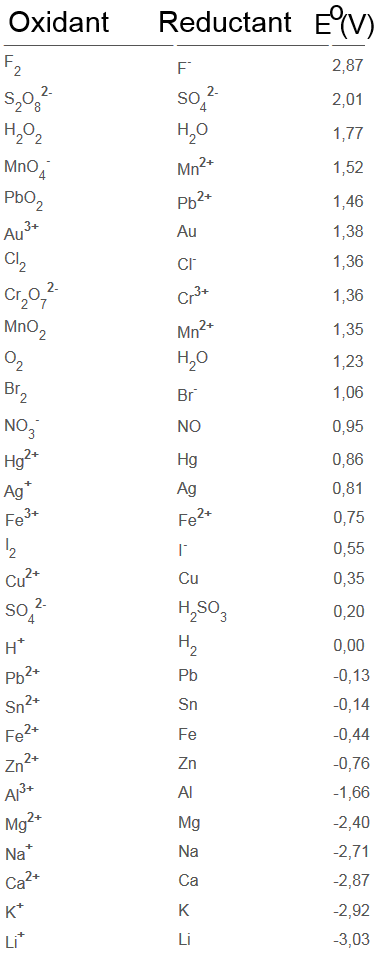Search:

# The oxidation reduction couples

## Example: The halogens and their anions

You see three halogens: chlorine $Cl_2$, bromine $Br_2$ and iodine $I_2$ and also their corresponding anions: chloride $Cl^-$,bromide $Br^-$ and iodide $I^-$ in separated aqueous solutions. Be sure to note their colours!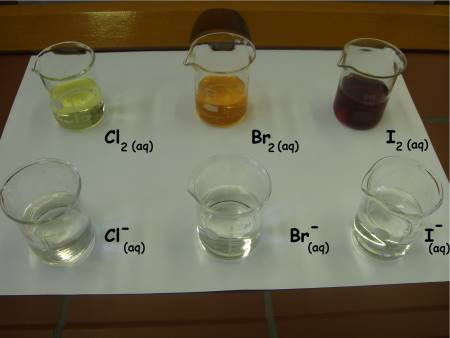Let's see which halogen reacts with which anion (nine reactions to try!):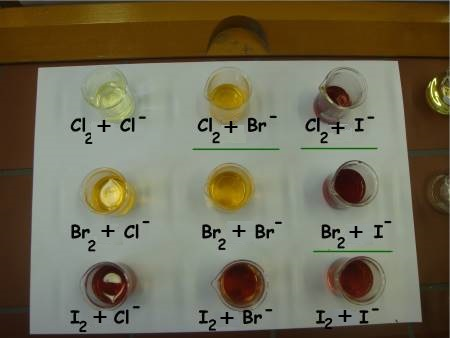We notice the following results: Chlorine and bromide: $Cl_2$ $+$ $2e^-$ $\longrightarrow$ $2Cl^-$ $2Br^-$ $+$ $2e^-$ $\longrightarrow$ $Br_2$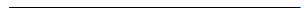$Cl_2$ (jaune)$\;+\;2Br^-\longrightarrow\;$$Br_2(orange)\;+\;2Cl^- Chlorine and iodide: Cl_2 + 2e^- \longrightarrow 2Cl^- 2I^- + 2e^- \longrightarrow I_2Cl_2 (jaune)\;+\;2I^-\longrightarrow\;$$I_2$(brown)$\;+\;2Cl^-$ Bromine and iodide: $Br_2$ $+$ $2e^-$ $\longrightarrow$ $2Br^-$ $2I^-$ $+$ $2e^-$ $\longrightarrow$ $I_2$$Br_2$ (orange)$\;+\;2I^-\longrightarrow\;$$I_2$(brown)$\;+\;2Br^-$

## Interpretation

Symbolising each reaction by an arrow, we have :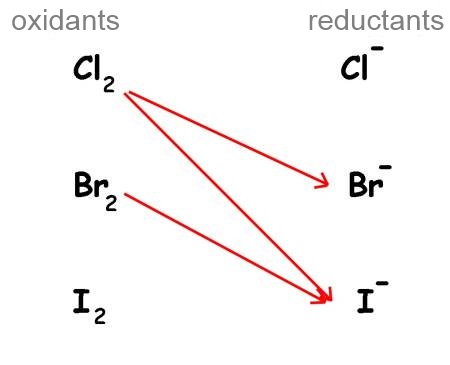- Oxidants(left) and reductants(right) are grouped by couples in a sort of table. - The strongest oxidant in this table is $Cl_2$ because he reacts with two reductants! - The strongest reductant in this table is $I^-$ because he reacts with two oxidants! - Every oxidant of the table can react with all the reductants situated below. - Every reductant of the table can react with all the oxidants situated above.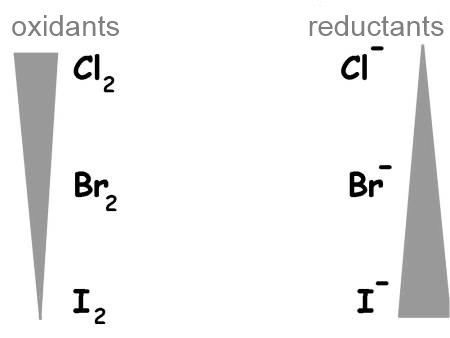## Generalisation

Oxidising agents and reducing agents are grouped in a table called redox table. As we have seen before, oxidants are situated on the left by decreasing strength from top to bottom, reductants to the right by increasing strength from top to bottom. Here are the most common couples: